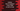# How to append a list, tuple or set to a set in python## Appending a list, tuple or set to a python set:

Python sets are used to store non-duplicate, unique elements. It is mutable. We can edit it and append data to it. set gives one method called add to add one single element to a set. Another method called update can be used to add multiple elements from another list, tuple or set. In this post, we will learn how to append items from a list, tuple or set to a python set.

### Definition of update:

update method is defined as below:

``given_set.update(param)``

Here, param can be a set, list, tuples or dictionary. This method returns nothing. It only adds the items to the set given_set.

### Example of adding list items to set in python:

Let’s take a look at the below example:

``````given_list = [1, 2, 3]
given_set = {4, 5, 6}

given_set.update(given_list)

print(given_set)``````

Here,

• given_list is a list of numbers
• given_set is a set with three numbers
• We are using update to append the values of given_list to given_set.
• The last line is printing the values of given_set which also includes the values of given_list.

If you run this program, it will give the below output:

``{1, 2, 3, 4, 5, 6}``Now, let’s try to add some duplicate items to the set.

``````given_list = [1, 2, 3]
given_set = {2, 3, 4, 5, 6}

given_set.update(given_list)

print(given_set)``````

If you run it, it will give the same output. Because, we can’t have duplicate values in a python set.

### Example of appending tuple to a set in python:

Let’s try to append a tuple to a set:

``````given_tuple = (1, 2, 3)
given_set = {2, 3, 4, 5, 6}

given_set.update(given_tuple)

print(given_set)``````

Here,

• given_tuple is a tuple with three numbers
• given_set is a set with five numbers.
• We are using update to add the numbers of tuple in the set.

If you run this program, it will print the same output.

### Example of appending set to a set in python:

The below example appends the numbers of one set to another set:

``````first_set = {1, 2, 3}
second_set = {2, 3, 4, 5, 6}

first_set.update(second_set)

print(first_set)``````

It is appending the values of second_set to first_set. It prints the same output.